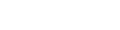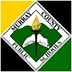Math

Progress monitoring assessments measure students’ response to intervention and progress throughout the year.

• If the student’s score is above the 50th percentile line, the teacher can say, “This particular skill area is not an issue.”

• If the student’s score is between the 10th and 50th percentile, the teacher can say, “This particular skill is an area of weakness” and then select that measure for Progress © University of Oregon 12 Monitoring.

• If the student’s score is below the 10th percentile, then the teacher knows: − there may be reason to suspect an even earlier skill deficit (

Optimally, the math tests should be used no more than once every three weeks for monitoring progress.

• Focus on one particular measure type at a time, transitioning to the next measure type after all ten Progress Monitoring forms have been used for a given type, orThe Number and Operations measure is included at all grade levels and addresses basic operations (addition, subtraction, multiplication, division) appropriate for each grade level. The test is untimed and can be administered via paper-and-pencil or online.

The Number and Operations measure is included at all grade levels and addresses basic operations (addition, subtraction, multiplication, division) appropriate for each grade level. The test is untimed and can be administered via paper-and-pencil or online.The Progress Monitoring tests typically take between 8 to 15 minutes to complete. The total score is the number of items that the student correctly answers. A sample from one of the kindergarten Number and Operations measures is shown in (Figure 15)

A specific Geometry measure is included in the Math measures for Kindergarten and grades one and three. The measure includes items that vary in complexity (for example, identification of shapes to calculation of perimeter). It is an untimed test that can be administered online or via paper-and-pencil. It takes approximately 8 to 15 minutes to complete. The total score is the number of items that the student correctly answers (Figure ).

The Measurement measure is included in the Kindergarten, second and fourth grade measures. It is designed to include items that assess various aspects of measurement (e.g., linear measurement, calculation of area, estimation, telling time). It is an untimed test that takes approximately 8 to 15 minutes to complete. The test may be administered online or via paperand-pencil. The total score is the number of items the student correctly answers (Figure 17)

The Number and Operations and Algebra measure is designed to assess basic algebraic knowledge utilizing all four operations (addition, subtraction, multiplication, division). The measures are available for grades 1–4 and 7, and take about 8 to 15 minutes to complete. These untimed measures are available online and paper-and-pencil. The total score is comprised of the total number of items that the student answered correctly (Figure 18).P. O. Box 40